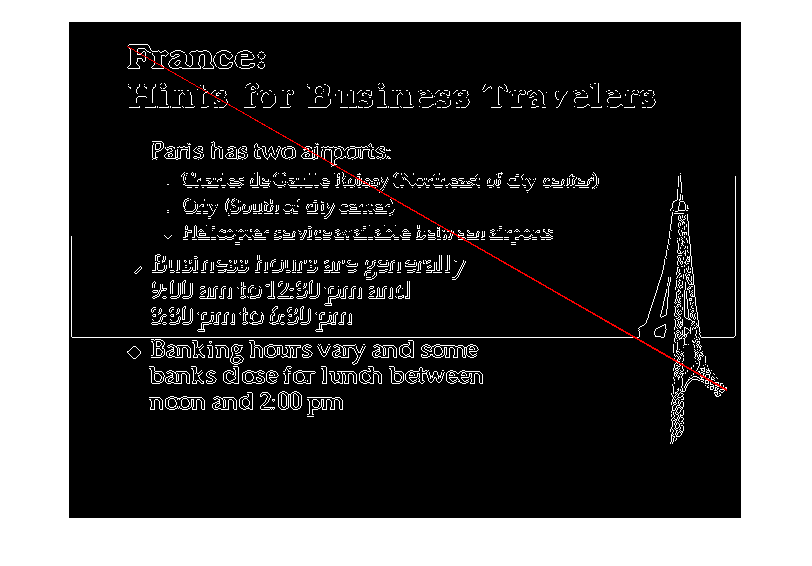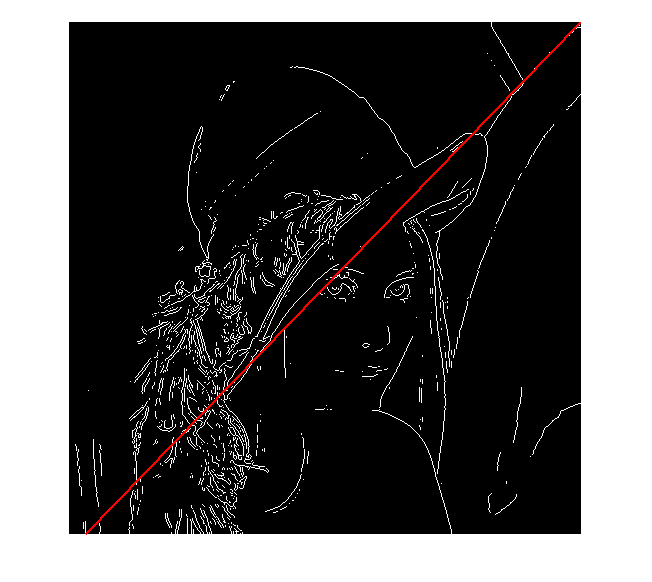#### How to detect the maximum pixel distance in a binary image

February 10, 2014In a recently posted question @StackOverflow a user asked how to find the maximum Euclidean distance in a binary image produced by Canny edge detection using MATLAB. The original post is here. I report my response here just for archival purposes.

Provided that you have a binary edge image, you need to think of white pixels as points in a 2D space and look for the coordinates that correspond to the largest distance.
MATLAB can easily do this using the pdist2 function with some extra parameters.
Here is a piece of code to do the trick:

```img = imread( 'image.bmp');
[ y, x] = find( img);
points = [ x y];
[d,idx] = pdist2( points, points, 'euclidean', 'Largest', 1);
idx1 = idx( d==max(d));
[~,idx2] = find(d==max( d));

p={};
for i=1:length(idx1)
p{end+1} = [ points(idx1(i),1), points(idx1(i),2), points(idx2(i),1), points(idx2(i),2)];
end
```

First you need to know where those white pixels are, so you initiate with a find function to get the coordinates. Then you use pdist2, which (in this case) returns the largest distances in a row matrix d and the corresponding indices in the row matrix i. Then you just need to check where is the maximum value in the largest distances to get the indices of the points with the maximum distance (these points are in a cell matrix of points p). This code captures all the possible maximum distance pixels, which could be more than a pair (ie. there could be pixel pairs with the same maximum distance). Of course, one may change the ‘euclidean’ parameters in the pdist2 function to get distances based on other metrics.

In order to keep only one copy of each line produced by the code the following code should be included:

```pp=[];
for i=1:numel(p)
for j=i+1:numel(p)
if prod( double( [p{i}(3:4),p{i}(1:2)] == p{j}))
pp(end+1)=j;
end
end
end
j=1;
ppp={};
for i=1:numel(p)-numel(pp)
if j<=numel(pp) && i~=pp(j)
ppp{end+1}=p{i};
j=j+1;
end
end
p = ppp;
```

And if there is a need to display the result, another piece of code is needed as follows:

```figure;
imshow( img);
hold on;
for i=1:numel(p)
line( [ p{i}(1), p{i}(3)], [p{i}(2), p{i}(4)]);
hdl = get(gca,'Children');
set( hdl(1),'LineWidth',2);
set( hdl(1),'color',[1 0 0]);
end
hold off;
```

Here are some example result images: